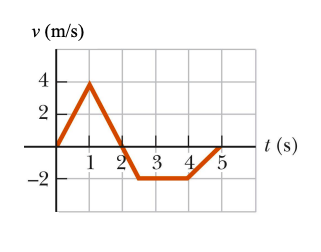# Position, Velocity and Acceleration#

The figure shows the velocity-versus-time graph for a particle moving along the x-axis. Its position at $$t$$ = 0 s is $$m$$.## Part 1#

What is the particles velocity at $$t$$ = 3 $$s$$? If the answer is undefined, enter 100.

Please enter in a numeric value in $$m$$.

## Part 2#

What is the particles position at $$t$$ = 3 $$s$$? If the answer is undefined, enter 100.

Please enter in a numeric value in $$m/s$$.

## Part 3#

What is the particles acceleration at $$t$$ = 3 $$s$$? If the answer is undefined, enter 100.

Please enter in a numeric value in $$\frac{m}{s^2}$$.